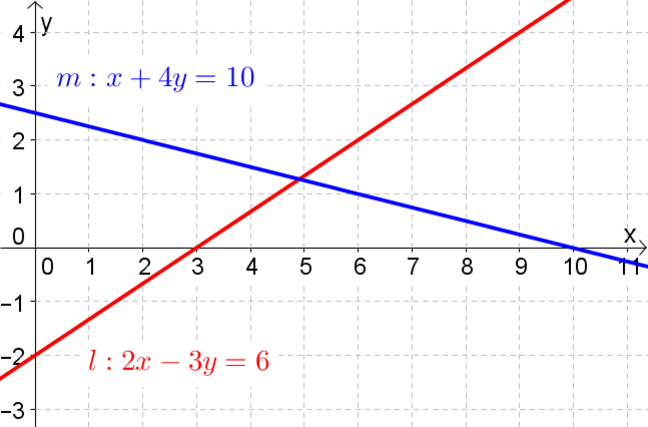## Want to keep learning?

This content is taken from the UNSW Sydney's online course, Maths for Humans: Linear, Quadratic & Inverse Relations. Join the course to learn more.
1.15

## UNSW SydneyIf lions can be tamed, then so too can lines

# Taming the algebraic duplicity of lines!

Lines are represented by linear equations. But there are a couple of options in how to present such linear equations, giving us two quite different ways of thinking about how the equation of a line relates to its geometrical form in the Cartesian plane.

In this lesson we will learn

• about the two different algebraic forms for a line

• the significance of the slope and the intercepts of a line.

## Two ways of writing a straight line

Every line can be written in the form

$\Large{ax+by=c}$

for some numbers $$\normalsize{a,\;b,\;c}$$. In fact we should require that $$\normalsize{a}$$ and $$\normalsize{b}$$ are not both zero. This form has the advantage of being the least restrictive.

Every non-vertical line can be written in the form

$\Large{y=mx + b.}$

This is called the slope-intercept form of a line. This form emphasises the slope $$\normalsize{ m}$$, also called the gradient , along with the $$\normalsize{y}$$-intercept $$\normalsize{b}$$ of the line.

Q1 (M): What line cannot be written in slope-intercept form?

So we have seen two different ways of writing the same line. For example the line $$\normalsize{l:\; 2x-3y=6}$$ can also be written as $$\normalsize{l:\; y=(2/3)x-2}$$. The line $$\normalsize{m: \; y=(-1/4)x+5/2}$$ can also be written as $$\normalsize{m:\; x+4y=10}$$.

Q2 (E): What is the slope of the line $${\normalsize m: x+4y = 10}$$?

Q3 (E): Find the $$\normalsize{x}$$- and $$\normalsize{y}$$-intercepts of the line $$\normalsize{l:\; 2x-3y=6}$$ pictured below.## Manipulating general equations

It is often instructive to work with abstract equations rather than special cases. Having letters represent variable quantities, such as the coefficients of $$\normalsize{x}$$ and $$\normalsize{y}$$ in the equation of a line can lead to more insight into what is really going on.

For example if we multiply two numbers, say $$\normalsize{3}$$ and $$\normalsize{4}$$, then the result is $$\normalsize{12}$$, but the original two numbers are more or less invisible. However if we multiply two variables, say $$\normalsize{a}$$ and $$\normalsize{b}$$, then the result is $$\normalsize{ab}$$ and we can see more clearly where this came from.

So the general line

$\Large{ax+by=c}$

can be rearranged first as $$\normalsize{by=-ax+c}$$ and, if $$\normalsize b \neq 0$$, then as

$\Large{y=-\frac{a}{b}x+\frac{c}{b}.}$

Going the other way, $$\normalsize{y=mx+b}$$ could be rearranged as $$\normalsize{mx-y=-b}$$.

Q4 (M): Find the slope and $$\normalsize{x}$$- and $$\normalsize{y}$$- intercepts of $$\normalsize{l:\; ax+by=c}$$.

Q5 (M): Find the $$\normalsize{x}$$- and $$\normalsize{y}$$- intercepts of $$\normalsize{k:\; y=mx+b}$$.

A1. The line with equation $$\normalsize{x=k}$$ cannot be written in the $$\normalsize{ y=mx+b}$$ form.

A2. The line can also be written as

${\Large y = -\frac{1}{4}x + \frac{5}{2}.}$

We can read off the slope from this form of the line: $${\normalsize m=-1/4}$$.

A3. To find the $$x$$-intercept of the line $$\normalsize{l:\; 2x-3y=6}$$ we set $$\normalsize{y=0}$$. This gives the equation $$\normalsize{l:\; 2x=6}$$ so that $$\normalsize{x=3}$$. To find the $$\normalsize{y}$$-intercept we set $$\normalsize{x=0}$$, which gives the equation $$\normalsize{l:\; -3y=6}$$ so that $$\normalsize{y=-2}$$. Have a look at the picture of the line to convince yourself that these values are correct.

A4. The slope of this line is $$\normalsize m=-a/b$$. The $$\normalsize{x}$$-intercept of $$\normalsize{l:\; ax+by=c}$$ is what you get when you set $$\normalsize{y=0}$$ and solve for $$\normalsize{x}$$, namely $$\normalsize{x=c/a}$$. The $$\normalsize{y}$$-intercept is what you get when you set $$\normalsize{x=0}$$ and solve for $$\normalsize{y}$$, namely $$\normalsize{y=c/b}$$.

A5. The $$\normalsize{x}$$-intercept of $$\normalsize{k:\; y=mx+b}$$ is $$\normalsize{-b/m}$$ and the $$\normalsize{y}$$-intercept is $$\normalsize{b}$$. Note that the $$\normalsize{y}$$-intercept is the easy one to find.